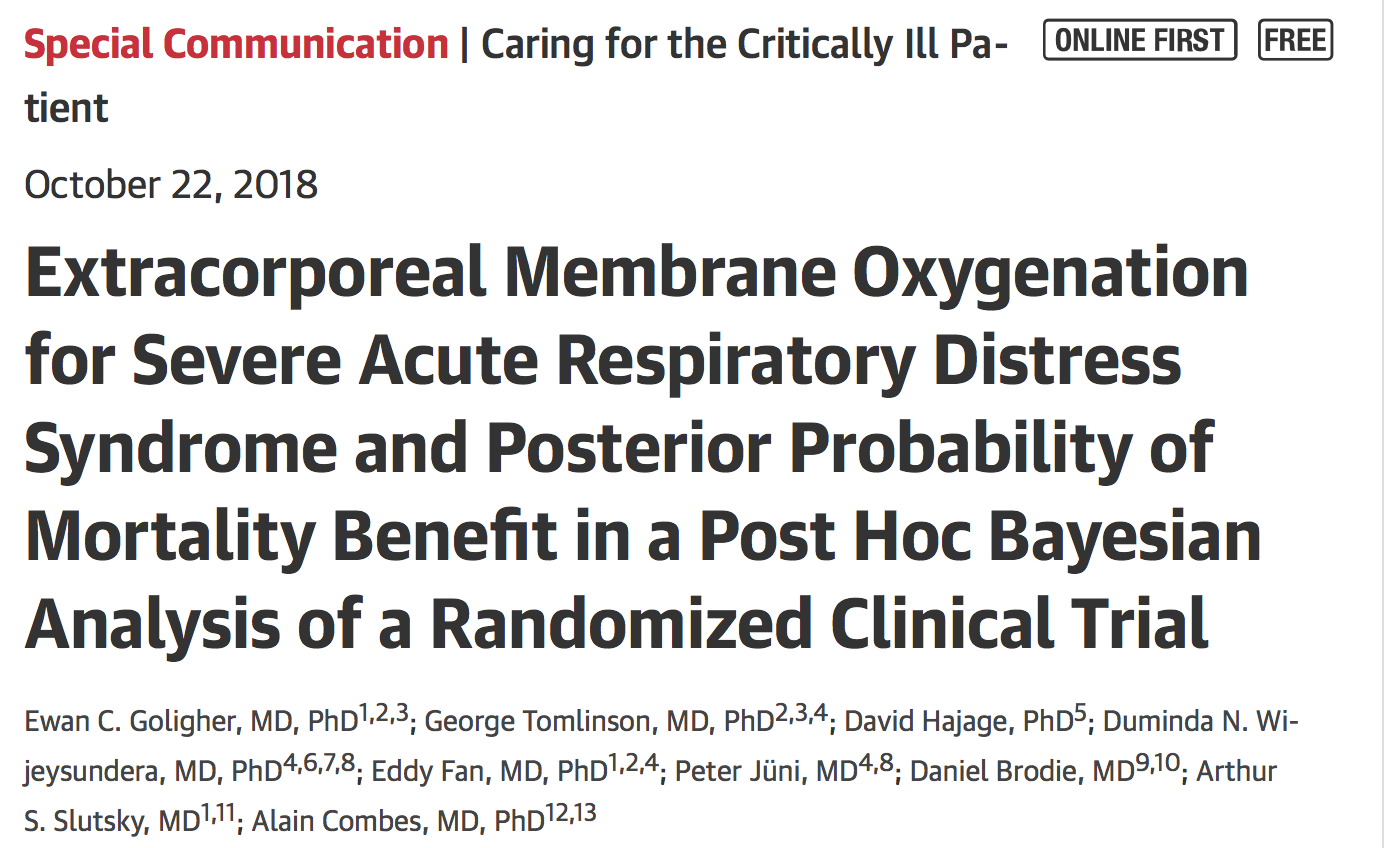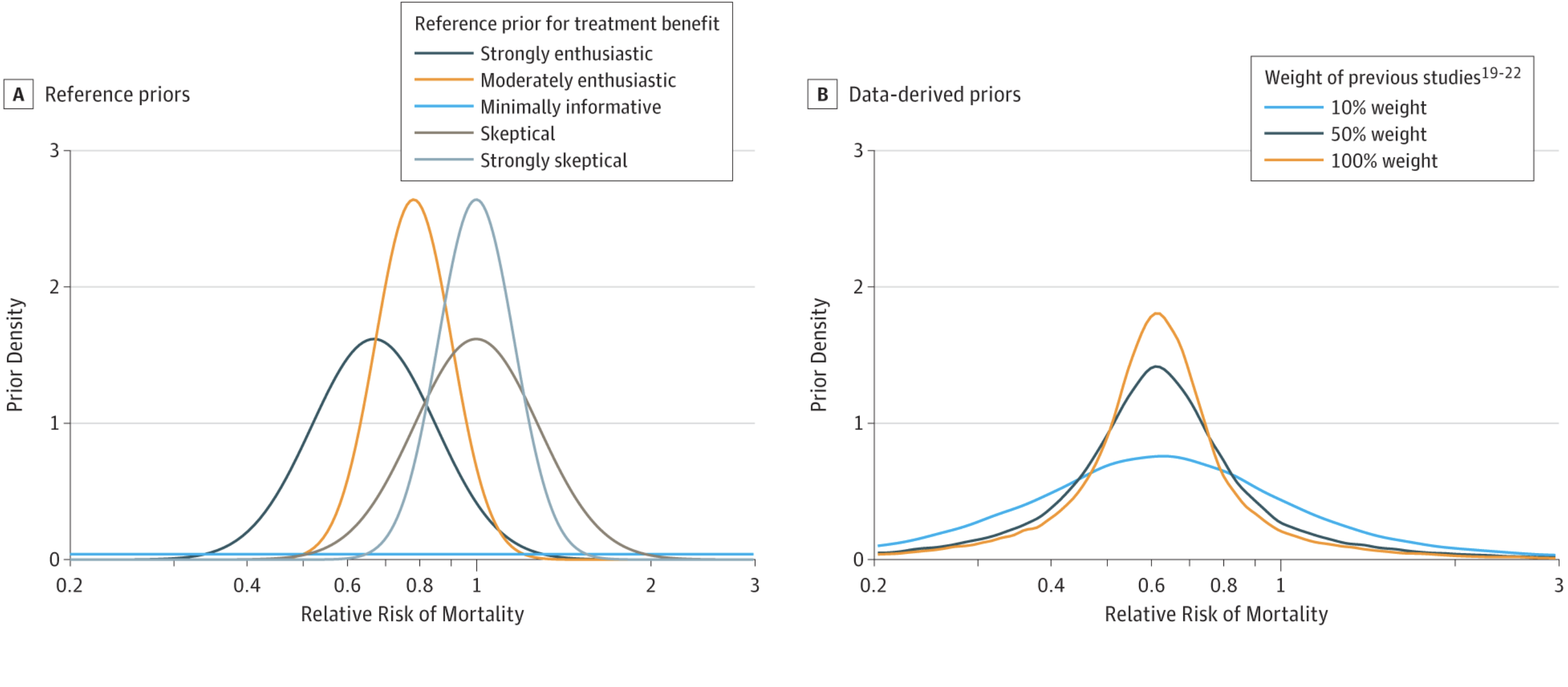# A post-hoc bayesian analysis of the EOLIA studyKey Points

Question  Can Bayesian analysis clarify the interpretation of clinical trial results?

Findings  In a post hoc Bayesian analysis of the recent EOLIA (Extracorporeal Membrane Oxygenation [ECMO] to Rescue Lung Injury in Severe ARDS) trial, the posterior probability of mortality benefit (relative risk <1) ranged between 88% and 99% given a range of prior assumptions reflecting varying degrees of skepticism and enthusiasm regarding previous evidence for the benefit of ECMO. Probabilities varied according to the definition of minimum clinically important mortality benefit; for example, the posterior probability of relative risk less than 0.67 ranged between 0% and 48% given the same range of prior assumptions.

Meaning  Information about the posterior probability of treatment effect provided by Bayesian analysis may help clarify the interpretation of clinical trial findings.

Abstract

Importance  Bayesian analysis of clinical trial data may provide useful information to aid in study interpretation, especially when trial evidence suggests that the benefits of an intervention are uncertain, such as in a trial that evaluated early extracorporeal membrane oxygenation (ECMO) for severe acute respiratory distress syndrome (ARDS).

Objective  To demonstrate the potential utility of Bayesian analyses by estimating the posterior probability, under various assumptions, that early ECMO was associated with reduced mortality in patients with very severe ARDS in a randomized clinical trial (RCT).

Design and Evidence  A post hoc Bayesian analysis of data from an RCT (ECMO to Rescue Lung Injury in Severe ARDS [EOLIA]) that included 249 patients with very severe ARDS who had been randomized to receive early ECMO (n = 124; mortality at 60 days, 35%) vs initial conventional lung-protective ventilation with the option for rescue ECMO (n = 125, mortality at 60 days, 46%). The trial was designed to detect an absolute risk reduction (ARR) of 20%, relative risk (RR) of 0.67. Statistical prior distributions were specified to represent varying levels of preexisting enthusiasm or skepticism for ECMO and by Bayesian meta-analysis of previously published studies (with downweighting to account for differences and quality between studies). The RR, credible interval (CrI), ARR, and probability of clinically important mortality benefit (varying from RR less than 1 to RR less than 0.67 and ARR from 2% or more to 20% or more) were estimated with Bayesian modeling.

Findings  Combining a minimally informative prior distribution with the findings of the EOLIA trial, the posterior probability of RR less than 1 for mortality at 60 days after randomization was 96% (RR, 0.78 [95% CrI, 0.56-1.04]); the posterior probability of RR less than 0.67 was 18%, the probability of ARR of 2% or more was 92%, and the probability of ARR of 20% or more was 2%. With a moderately enthusiastic prior, equivalent to information from a trial of 264 patients with an RR of 0.78, the estimated RR was 0.78 (95% CrI, 0.63-0.96), the probability of RR less than 1 was 99%, the probability of RR less than 0.67 was 8%, the probability of ARR of 2% or more was 97%, and the probability of ARR of 20% or more was 0%. With a strongly skeptical prior, equivalent to information from a trial of 264 patients with an RR of 1.0, the estimated RR was 0.88 (95% CrI, 0.71-1.09), the probability of RR less than 1 was 88%, the probability of RR less than 0.67 was 0%, the probability of ARR of 2% or more was 78%, and the probability of ARR of 20% or more was 0%. If the prior was informed by previous studies, the estimated RR was 0.71 (95% CrI, 0.55-0.94), the probability of RR less than 1 was 99%, the probability of RR less than 0.67 was 48%, the probability of ARR of 2% or more was 98%, and the probability of ARR of 20% or more was 4%.

Conclusions and Relevance  Post hoc Bayesian analysis of data from a randomized clinical trial of early extracorporeal membrane oxygenation compared with conventional lung-protective ventilation with the option for rescue extracorporeal membrane oxygenation among patients with very severe acute respiratory distress syndrome provides information about the posterior probability of mortality benefit under a broad set of assumptions that may help inform interpretation of the study findings.

Find the article here !

Last updated: 24/10/2018